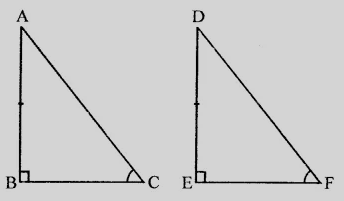# In two right triangles one side an acute angle of one are equal to the corresponding side and angle of the other. Prove that the triangles are congruent.

Given:

In two right triangles one side an acute angle of one are equal to the corresponding side and angle of the other.

To do:

We have to prove that the triangles are congruent.

Solution:Let in triangles $ABC$ and $DEF$,

$\angle B = \angle E = 90^o$

$\angle C = \angle F$

$AB = DE$

Therefore, by AAS axiom,

$\triangle ABC \cong \triangle DEF$

Hence proved.

Updated on: 10-Oct-2022

33 Views